Download Presentation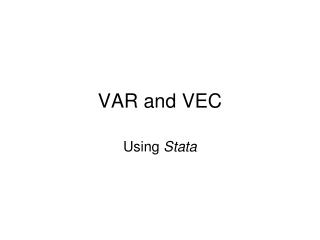VAR and VEC

# VAR and VEC - PowerPoint PPT Presentation

VAR and VEC. Using Stata. VAR: Vector Autoregression. Assumptions: y t : Stationary K-variable vector v : K constant parameters vector A j : K by K parameters matrix, j=1,…,p u t : i.i.d.( 0 , S ) Trend may be included: d t, where d is K by 1 Exogenous variables X may be added.I am the owner, or an agent authorized to act on behalf of the owner, of the copyrighted work described.
Download Presentation## VAR and VEC

An Image/Link below is provided (as is) to download presentation

Download Policy: Content on the Website is provided to you AS IS for your information and personal use and may not be sold / licensed / shared on other websites without getting consent from its author.While downloading, if for some reason you are not able to download a presentation, the publisher may have deleted the file from their server.

- - - - - - - - - - - - - - - - - - - - - - - - - - E N D - - - - - - - - - - - - - - - - - - - - - - - - - -
Presentation Transcript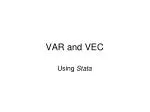### VAR and VEC

Using Stata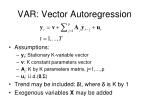VAR: Vector Autoregression
• Assumptions:
• yt: Stationary K-variable vector
• v: K constant parameters vector
• Aj: K by K parameters matrix, j=1,…,p
• ut: i.i.d.(0,S)
• Trend may be included: dt, where d is K by 1
• Exogenous variables X may be added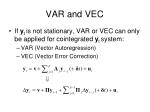VAR and VEC
• If yt is not stationary, VAR or VEC can only be applied for cointegrated yt system:
• VAR (Vector Autoregression)
• VEC (Vector Error Correction)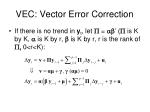VEC: Vector Error Correction
• If there is no trend in yt, let P = ab’ (P is K by K, a is K by r, b is K by r, r is the rank of P, 0<r<K):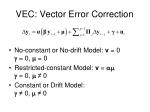VEC: Vector Error Correction
• No-constant or No-drift Model: v = 0 g = 0, m = 0
• Restricted-constant Model: v = amg = 0, m≠ 0
• Constant or Drift Model: g≠ 0, m≠ 0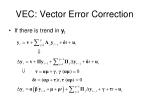VEC: Vector Error Correction
• If there is trend in yt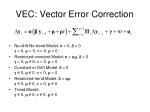VEC: Vector Error Correction
• No-drift No-trend Model: v = 0, d = 0 g = 0, m = 0, t = 0, r = 0
• Restricted-constant Model: v = am, d = 0 g = 0, m≠ 0, t = 0, r = 0
• Constant or Drift Model: d = 0 g≠ 0, m≠ 0, t = 0, r = 0
• Restricted-trend Model: d = arg≠ 0, m≠ 0, t = 0, r≠ 0
• Trend Model:g≠ 0, m≠ 0, t≠ 0, r≠ 0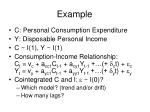Example
• C: Personal Consumption Expenditure
• Y: Disposable Personal Income
• C ~ I(1), Y ~ I(1)
• Consumption-Income Relationship:Ct = vc + acc1Ct-1 + acy1Yt-1 +…(+ dct) + ecYt = vy + ayc1Ct-1 + ayy1Yt-1 +…(+ dyt) + ey
• Cointegrated C and I: e ~ I(0)?
• Which model? (trend and/or drift)
• How many lags?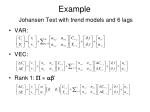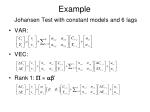ExampleJohansen Test with constant models and 6 lags
• Constant model: v = am + g (a 0,g0)
• Restricted-constant model: v = am (a 0)
• No-constant model: v = 0ExampleJohansen Test with trend models and 6 lags
• Trend model:d = ar+t g≠ 0, m≠ 0, t≠ 0, r≠ 0
• Restricted trend model: d = ar g≠ 0, m≠ 0, t = 0, r≠ 0
• No trend or constant model: d = 0 g≠ 0, m≠ 0, t = 0, r= 0Example VEC estimated models with 6 lags
• Empirical Model: 1948q3 – 2012q3 (N=257)
• No-constant: LL=1766.15 (K=23)
• Restricted-constant: LL=1771.47 (K=24)
• Restricted-trend: LL=1774.15 (K=27)VEC Forecasting Estimated Models: 1948q3 – 2009q4
• No-constant model, 6 Lags (LL=1684.37, 23 parameters, N=246)
• Restricted-constant model, 6 lags (LL=1689.23, 24 parameters, N=246)
• Restricted-trend model, 6 lags (LL = 1691.9, 27 parameters, N=246)